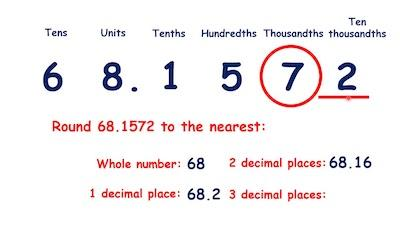# How To Round Off Decimal Numbers – Ultimate Guide

Just like you can round off whole numbers, you can round off decimal numbers as well.

Ultimately, decimal numbers are rounded off to estimate an answer quickly and easily. It’s important to keep in mind that decimal numbers can be rounded off to the nearest integer or whole number, tenths, hundredths, thousandths etc.

Discover how to round off numbers.

## Rounding Off Decimal Numbers To The Nearest Integer

When you are looking to round off decimal numbers to the nearest integer or whole number, you just need to follow the next rules:

#1: You need to identify the number to be rounded off.

#2: You should mark the digit in ones place.

#3: The first digit to the right of the decimal, or in tenths place, is checked.

#4: If the tenths digit is less than or equal to 4, then the number in the ones place is rounded off to a whole number. Similarly, if the tenths digit is greater than or equal to 5, add 1 digit to the number in the ones place.

#5: Drop all digits after the decimal point to result in the desired whole number.

Learn how to multiply decimals.

## Rounding Off Decimal Numbers To The Nearest Tenths

When you are looking to round off decimal numbers to the nearest tenths, the procedure is basically the same. However, here are the rules you should follow:

#1: You need to identify the number to be rounded off.

#2: Now, you need to mark the digit in the tenths place.

#3: You now need to check the digit in the hundredths place.

#4: If the hundredths digit is less than or equal to 4, then the number in the tenths digit remains the same. Similarly, if the hundredths digit is greater than or equal to 5, add 1 digit to the number in the tenths place.

#5: Drop all digits to the right of the tenths column to result in the desired number.

This is how you add and subtract decimals.

## Rounding Off Decimal Numbers To The Nearest Hundredths

Just like we explained above, the first step is to identify the required number. The digit in the hundredths place is marked. Check whether the digit in the thousandths’ place is 0, 1, 2, 3, 4 or 5, 6, 7, 8, 9. Round off the number to the nearest hundredths and drop the other numbers to the right of the hundredths place.

## Round Off Decimal Numbers – Practical Examples

Practical Example #1: Round off 975.6539 to the nearest whole number

As you can see, the number in the tenths place is 6. So, you will need to  add one digit to the ones digit and drop all the numbers after the decimal point.

975.6539 → 976

Practical Example #2: Round off the following number to the nearest tenths: 845.324

In this case, the hundredths digit is 2. So, you’ll need to drop all the digits after hundredths place.

845.324 → 845.30

Discover how to convert unlike decimals to like decimals.

Practical Example #3: The length of a road is 27.56 km. What is the length to the nearest km?

In this case, you will need to round this decimal to the nearest whole number by simply following the above-mentioned rules:

27.56 → 28 km